# GMAT Math : Arithmetic Mean

## Example Questions

← Previous 1 3 4 5 6

### Example Question #1 : Calculating Arithmetic Mean

Salaries for employees at ABC Company: 1 employee makes $25,000 per year, 4 employees make$40,000 per year, 2 employees make $50,000 per year and 5 employees make$75,000 per year.

What is the average (arithmetic mean) salary for the employees at ABC Company?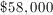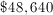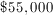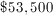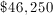Explanation:

The average is found by calculating the total payroll and then dividing by the total number of employees.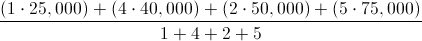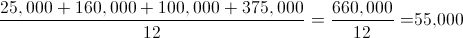### Example Question #1 : Arithmetic Mean

A bowler had an average (arithmetic mean) score of 215 on the first 5 games she bowled. What must she bowl on the 6th game to average 220 overall?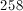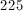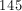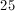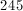Explanation:

For the first 5 games the bowler has averaged 215. The equation to calculate the answer is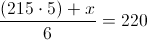whereis the score for the sixth game. Next, to solve for the score for the 6th game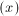multiply both sides by 6: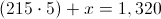which simplifies to: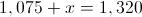After subtracting 1,075 from each side we reach the answer: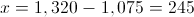### Example Question #1 : Calculating Arithmetic Mean

Ashley averaged a score of 87 on her first 5 tests. She scored a 93 on her 6th test. What is her average test score, assuming all 6 tests are weighted equally?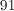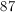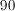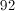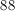Explanation:

We can't just average 87 and 93! This will give the wrong answer! The average formula is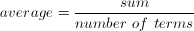.

For the first 5 tests,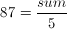. Then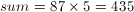.

Now combine that with the 6th test to find the overall average.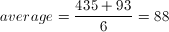### Example Question #471 : Arithmetic

Sabrina made $3,000 a month for three months,$4,000 the next month, and \$5,200 a month for the following two months. What was her average monthly income for the 6 month period?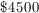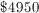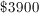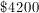Explanation: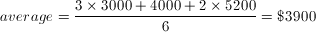### Example Question #5 : Arithmetic Mean

Luke counts the number of gummy bears he eats every day for 1 week: {39, 18, 24, 51, 40, 15, 23}. On average, how many gummy bears does Luke eat each day?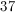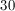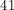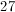Explanation: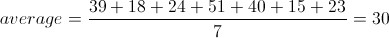### Example Question #21 : Descriptive Statistics

The average of 10, 25, and 70 is 10 more than the average of 15, 30, and x.  What is the missing number?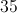Explanation:

The average of 10, 25, and 70 is 35: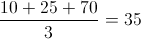So the average of 15, 30, and the unknown number is 25 or, 10 less than the average of 10, 25, and 70 (= 35)

so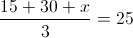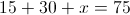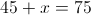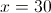### Example Question #1 : Arithmetic Mean

What is the average of 2x, 3x + 2, and 7x +4?

7x

4x + 2

3x + 4

not enough information

4

4x + 2

Explanation: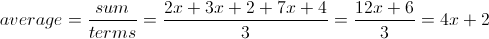### Example Question #1 : Arithmetic Mean

The average high temperature for the week is 85.  The first six days of the week have high temperatures of 89, 76, 92, 90, 80, and 84, respectively.  What is the high temperature on the seventh day of the week?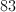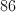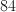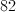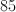Explanation: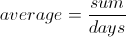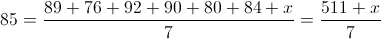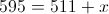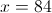### Example Question #9 : Arithmetic Mean

Jimmy's grade in his finance class is based on six equally-weighted tests.  If Jimmy scored 98, 64, 82, 90, 70, and 88 on the six tests, what was his grade in the class?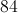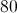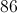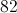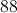Explanation: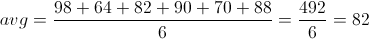### Example Question #1 : Arithmetic Mean

Sandra's grade in economics depends on seven tests - five hourly tests, a midterm, and a final exam. The midterm counts twice as much as an hourly test; the final, three times as much.

Sandra's grades on the five hourly tests are 84, 86, 76, 89, and 93; her grade on the midterm was 72. What score out of 100 must she achieve on the final exam so that her average score at the end of the term is at least 80?

She cannot achieve this average this term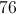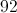Explanation:

This is a weighted mean, with the hourly tests assigned a weight of 1, the midterm assigned a weight of 2, and the final assigned a weight of 3. The total of the weights will be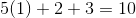.

If we letbe Sandra's final exam score, Sandra's final weighted average will be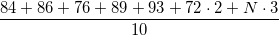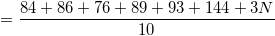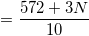For Sandra to get a final average of 80, then we set the above equal to 80 and calculate: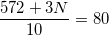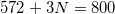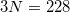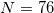← Previous 1 3 4 5 6

### All GMAT Math Resources